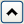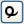You can include conditional expressions (called conditionals for short) in your formulas. These expressions let you build a sequence of clauses paired with result expressions. Constructing a sequence of clauses is the way you conditionally assign values to cells in a calculated column. Conditionals follow these rules:
 • When no clause is true, the Formula Editor evaluates the result expression that accompanies the else clause.
 • All result expressions in a conditional expression must evaluate to the same data type.
 • A missing term matches any data type.
 • By definition, expressions that evaluate as zero are false.
 • If an expression evaluates as missing, no clauses are executed and missing is returned. All other numeric expressions are true.
See the JSL Syntax Reference for details about syntax.
Note: Interpolate, Step, For, and While are most often used in conjunction with other commands to build a JSL script. You can use the Formula Editor to create and execute a script in that column, but this is not recommended because of dependencies and ambiguities that can result. Most often, scripts are stored as .jsl files, and can be saved with a data table as a table property. For details about table properties, see Table Panel in Get Started. For documentation of all scripting commands, see the JSL Syntax Reference.
Shows a single If condition with a missing expression and a missing then clause. Highlight either expr or then clause and enter a value. For example, to calculate count as a percentage of total when total is not 0, enter the conditional expression (using columns called count and total) in A Conditional Expression.
A Conditional Expression
To add a new condition to the If conditional, highlight then clause and click the insert button () on the Formula Editor keypad. Initially, this changes the existing else condition to an expr clause. Click the insert button again to add an else clause. Highlighting then or else and repetitively clicking the insert button changes the else to expr or adds a new expr clause.
To delete a clause, select the then clause above it and press the delete key on your keyboard or click the delete button () on the Formula Editor keypad.
After you select Match in the Formula Editor, a list appears with two options:
 • Select Add Match Arguments from Data, and clauses that correspond to all of the levels in your data are added automatically. Alternatively, hold down the SHIFT key, select Conditional, and then select Match. In Examples of Using the Match Function, the example on the left shows clauses that were added automatically.
 • Select Don’t Add so that you can add each clause individually. In Examples of Using the Match Function, the example on the right shows an empty clause, which you fill with the missing expressions.
Examples of Using the Match Function
In an automatically filled argument, you should highlight then clause, and then enter an expression. In an empty argument, you highlight either expr, value, or then clause, and then enter an expression. (Or, if you highlight an expression and click Match, the Formula Editor creates a new Match conditional, with the original highlighted expression as expr and nothing for the value and else clause.) Also, keep in mind that:
 • Match evaluates faster and uses less memory than an equivalent If because the variable is evaluated only once for each row in the data table. The If condition must evaluate the variable at each If clause for each row until a clause evaluates as true. See Comparison Functions, for a comparison of Match and If conditionals.
 • With If and Match, the Formula Editor searches down from the top of the sequence for the first true clause and evaluates the corresponding result expression. Subsequent true clauses are ignored.
An Example of Using the Match Function
Note: Match ignores trailing spaces and If does not.
Although Match returns missing for any missing values, you can also specifically match missing values.
Choose is a special case of Match in which the arguments of the condition are a sequence of integers starting at one. The value of clause replaces the match condition. An example of a Choose condition is shown in Example of a Choose Condition. With Choose, the Formula Editor goes directly to the correct choice clause and evaluates the result expression.
Example of a Choose Condition
When you highlight an expression and click Choose, the Formula Editor creates a new conditional expression with one clause. Use the insert () and delete () buttons on the keypad to add new clauses or remove unwanted clauses, as described previously for the If conditional.
Choose evaluates the choose expression and goes immediately to the corresponding result expression to generate the returned value. With Choose, you provide a choosing expression that yields sequential integers starting at 1 only once, and then you give a choice for each integer in the sequence.
Creating an And Function
Evaluates as 1 when either of its arguments is true. If both of its arguments are false, then the Or expression evaluates as 0. (See Evaluations of And and Or Expressions.) The formula in Creating an Or Function assigns males and all participants who are more than 13 years old to Group 1.
Creating an Or Function
The truth tables on the left in Evaluations of And and Or Expressions illustrate the results of the And ( & ) and Or (| ) functions when both arguments have nonmissing values that evaluate to true or false. The table on the right illustrates the result when either the left or right expression (call them a and b) or both have missing values.
Evaluations of And and Or Expressions
Evaluates as 1 when its argument is false. Otherwise, Not evaluates as 0. When you apply the Not function, use parentheses where necessary to avoid ambiguity. For example, !(weight==64) can be either true or false (either 1 or 0), but (!weight)==64 is always false (0) because Not can return only 0 or 1. Expressions such as !(weight==64) can also be entered as weight != 64.
Linearly interpolates the y-value between two points, x1, y1 and x2, y2 that corresponds to the arguments that you give. You can insert additional pairs of x, y arguments with the insert key. Interpolate finds the pair of x, y points that correspond to the x-value and completes the interpolation.
Is like Interpolate except that it returns the y-value corresponding to the greatest x-value less than or equal to the x and y arguments. That is, it finds the corresponding y for a given x from a step function rather than a linear fit between points. Like Interpolate, you can have as many x and y argument pairs as you want.
Example of Interpolate
Repeats the statements in the body argument as long as the while condition is true. The init and next control the iterations.
Repeatedly tests the expr condition and executes the body until expr is no longer true.
Break stops execution of a loop completely and continues to the statement following the loop. Continue ends the current iteration of a loop and begins the loop at the next iteration.
Both are used in For, While, and For Each Row loops.